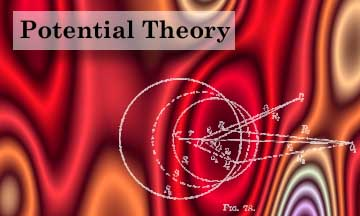Introduction

Potential Theory is a branch of theoretical physics that deals with phenomena having to do with attraction or the distribution of physical effects through space. It has additionally grown to be a lucrative branch of Mathematics as well, but the context of the information available on this page is confined to a physical interpretation involving the discussion of mutual Newtonian Attraction, i.e., gravitational attraction. Many other interesting applications of potential theory can be made in the areas of electromagnetism, heat propagation and nuclear physics.

The content found on this page largely deals with the classical theory involved. Potential theory is applied in studies of the gravitational attractions of the Earth and other terrestrial planetary bodies. Physical geodesy, a subdiscipline of geophysics, relies heavily on concepts arising in potential theory. The gravitational attraction of stars, galaxies and other large-scale celestial bodies is more properly treated in a study of astrophysics, which is not included on this web site.

Resources:

Hard Copy:

Potential Theory, specifically:

• Blakely, R., Potential Theory in Gravity and Magnetic Applications, Cambridge U. Press, Cambridge, 1995.
• Günter, N. M., Potential Theory and its Applications to Basic Problems of Mathematical Physics, F. Ungar, New York, 1967.
• Kellogg, O. D., Foundations of Potential Theory, F. Ungar Publishing, New York, 1929 (also in Dover reprint).
• MacMillan, W. D., The Theory of the Potential, Dover reprint, New York, 1958.
• Ramsey, A. S., Newtonian Attraction, Cambridge U. Press, Cambridge, 1981.
• Sigl, R., Introduction to Potential Theory, Abacus Press, Tunbridge Wells, Kent, UK, 1985.
• Sternberg, W. J. & T. L. Smith, The Theory of Potential and Spherical Harmonics, 2nd ed., U. of Toronto Press, Toronto, 1952.
• Wangerin, A., Theorie des Potentials und der Kugelfunktionen, 2 vols., Walter de Gruyter, Berlin, 1922.

• Related Mathematical Texts:

• Barton, G., Elements of Green's Functions and Propagation: Potentials, Diffusion and Waves, Oxford U. Press, Oxford, 1989.
• Bland, D. R., Solutions of Laplace's Equation, The Free Press, Glencoe, IL, 1961.
• Borisenko, A. I. & I. E. Tarapov, Vector and Tensor Analysis with Applications, Dover, New York, 1979.
• Byerly, W. E., An Elementary Treatise on Fourier Series and Spherical, Cylyndrical, and Ellipsoidal Harmonics with Applications to Problems in Mathematical Physics, Dover reprint, New York, 1893.
• Hobson, E. W., Spherical and Ellipsoidal Harmonics, Cambridge Univ. Press, Cambridge, 1931.
• Lebedev, N. N., Special Functions & Their Applications, Dover, New York, 1972.
• Lense, J., Kugelfunktionen, Akademische Verlagsgesellschaft, Leipzig, 1950.
• MacRobert, T. M., Spherical Harmonics, 3rd ed.,Pergamon Press, Oxford, 1967.
• Wangerin, A., Theorie des Potentials und der Kugelfunktionen, Walter de Gruyter, Berlin, 1922.

• Contents from Selected books:

 Contents of Kellogg's Foundations of Potential Theory Contents of MacMillan's Theory of the Potential The Force of Gravity Fields of Force The Potential The Divergence Theorem Properties of Newtonian Potentials at Points of Free Space Properties of Newtonian Potentials at Points Occupied by Masses Potentials as Solutions of Laplace's Equation; Electrostatics Harmonic Functions Electric Images; Green's Functions Sequences of Harmonic Functions Fundamental Existence Theorems The Logarithmic Potential The Attraction of Finite Bodies The Newtonian Potential Function Vector Fields. Theorems of Green and Gauss The Attractions of Surfaces and Lines Surface Distributions of Matter Two-Layer Surfaces Spherical Harmonics Ellipsoidal Harmonics

 Contents of MacRobert's Spherical Harmonics Contents of Hobson's Spherical & Ellipsoidal Harmonics Fourier Series Conduction of Heat Transverse Vibrations of Stretched Strings Spherical Harmonics: The Hypergeometric Function The Legendre Polynomials The Legendre Functions The Associated Legendre Functions of Integral Order Applications of Legendre Coefficients to Potential Theory Potentials of Spherical Shells, Spheres and Spheroids Applications to Electrostatics Ellipsoids of Revolution Eccentric Spheres Clerk Maxwell's Theory of Spherical Harmonics Bessel Functions Asymptotic Expansions and Fourier-Bessel Expansions Application of Bessel Functions The Hypergeometric Function Associated Legendre Functions of General Order The Transformation of Laplace's Equation The Solution of Laplace's Equation in Polar Coordinates The Legendre's Associated Functions Spherical Harmonics Spherical Harmonics of General Type Approximate Values of the Generalized Legendre's Functions Representation of Functions by Series The Addition Theorems for General Legendre's Functions The Zeros of Legendre's Functions and Associated Functions Harmonics for Spaces Bounded by Surfaces of Revolution Ellipsoidal Harmonics